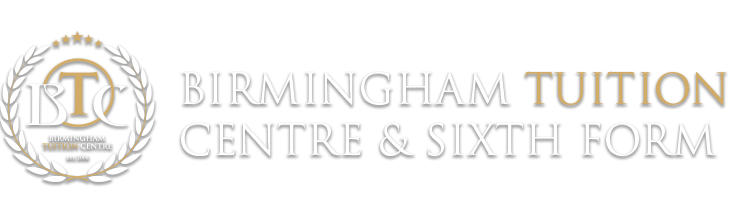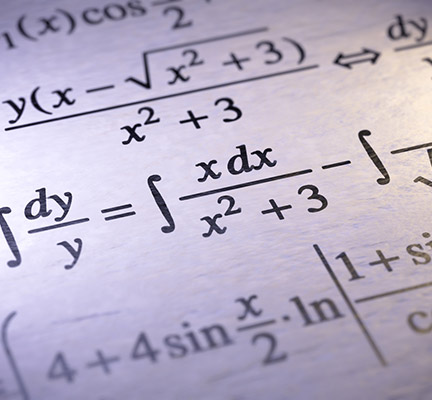## A Level Mathematics“For the things of this world cannot be made known without knowledge of mathematics.”Roger Bacon

A-level Mathematics is a prestigious subject that is highly regarded by the majority of Universities as it enables students to establish a wide range of skills. Students can use this A-Level to progress further into a range of professions including Teaching, Engineering, Accounting and Finance, Economics and Medicine.

Here at BTC, we encourage our AS and A2-Level students to reinforce their mathematical skills by comprehending mathematical problems and processes and developing their understanding of these concepts in a confident way. We also enable them to recognise how different situations are represented mathematically.

We recognise that Mathematics is a challenging A-level and in view of this we enable our students to appreciate a range of mathematical techniques that are used to solve difficult, unstructured problems.

The following modules are assessed at AS-Level:

 Module Exemplar Content Exemplar Questions Expectations: Core Maths 1 -Surds -Coordinate geometry of straight lines and circles -Quadratic functions -Polynomials -Simultaneous equations -Differentiation and Integration · Find the coordinates of the points on the curve y= X3 -9x + 5 where the gradient is equal to 3 · Given that f’(x) = (x-1)2 and f(0) = 2. Find f(x) -Students should be able to: ·Expand brackets ·Solve linear equations ·Understand and comprehend Pythagoras’ Theorem ·Appreciate and use Trigonometry as a tool in problem solving
 Core Maths 2 -Indices -Further Differentiation -Further integration and the Trapezium rule -Exponentials and logarithms -Geometric Series Factorials and binomial expansions -Simple transformation of graphs · Describe the geometrical transformation that maps the graph of y=1 – x5 onto the graph of y=1 + X5 ·Students are expected to have a sound understanding of C1. Statistics 1 -Numerical Measures -Probability -Binomial Distribution -Confidence Intervals -Correlation -Regression · Calculate the product moment correlation coefficient between x & y Sxx=2.41 Syy=3.56 Sxy=1.87 · Students are expected to know a wide range of mathematical formulae.

The following modules are assessed at A2-Level:

 Module Content Exemplar Questions Core Maths 3 -Functions – Transformations of graphs and the modulus function -Inverse trigonometric functions and secant, cosecant and cotangent -The number e and calculus -Further differentiation -Volume of revolution and numerical integration · Describe geometrically how the curve y=8x + 1 can be transformed into the curve y = 2x by a sequence of transformations · Students are expected to have a sound understanding C1 and C2. Core Maths 4 -Binomial series expansion -Rational functions and division of polynomials -Partial fractions and applications -Parametric equations -Further trigonometry with integration -Exponential growth and decay -Differential Equations -Vector equations of lines · Find the distance between the points (-5, 3, 7) and (-2,-2,6) · Students are expected to have a sound understanding C1, C2 and C3. Mechanics 1 -Mathematical models in mechanics -Vectors and their application in mechanics -Kinematics of a particle -Statics of a particle -Dynamics of a particle of moving in a straight line or plane -Moments · A cable raising a load with an acceleration of 1.5 m s -2 has a tension of 15kN. Find the mass of the load · Students are expected to have a sound understanding of C1.